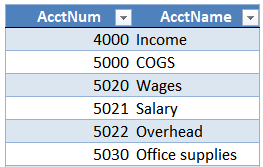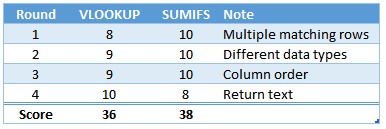# Battle of Heavyweights: Round 4Welcome to round 4. In this round, we want to explore the issue of return values. Specifically, the data types that our two heavyweights can return. Can they return text values? Numbers? So, that’s the idea … let’s jump right in. Round 4 … ding!

## Round 4

We have some account numbers, but we also need the account names. We have a worksheet that looks like this:The lookup table (Table1) looks like this:We want to use formulas to retrieve the account names from the lookup table.

First, we’ll try VLOOKUP.

## VLOOKUP

We write the following formula into C8:

`=VLOOKUP(B8, Table1, 2, 0)`

We fill the formula down, and bam:Works just fine. So, what does this tell us about VLOOKUP? It can return text. We’ve seen in previous rounds that it can return numbers as well. So, VLOOKUP can return text and numbers.

So far so good, now let’s try again with SUMIFS.

## SUMIFS

We write the following formula into D8:

`=SUMIFS(Table1[AcctName], Table1[AcctNum], B8)`

We fill it down, and … hmmm:A bunch of 0’s. So, what does this tell us about SUMIFS? It returns numbers, not text strings. Remember, it is a conditional summing function. It returns a sum … a number.

## Analysis

The point of this post is:

VLOOKUP can return text and numbers; SUMIFS returns a number

So, if we need to retrieve a text value, which function will we use? Yes … VLOOKUP! So, VLOOKUP wins the round!

We award 10 points to VLOOKUP and 8 points to SUMIFS.Updated stats …So, when the value we want to return is a number, we can consider SUMIFS … but when the value we want to return a text string, we’ll roll with VLOOKUP (or XLOOKUP if we have it)

Sample file: Round4.xlsx

Get a quick email notice when a new Excel article is available

• This field is for validation purposes and should be left unchanged.